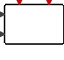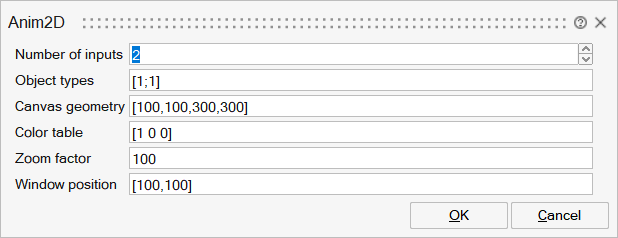# Anim2D

This block creates simple 2D animations of basic geometrical forms.SignalViewers

## Description

The animation block is used to animate different types of graphical objects in a Figure (on a canvas). Each input represent an object or a set of objects. An object type is associated with each input defining the geometrical form of the object(s).

Polylines. Type 1 is a closed polyline and the input is a Nx2 matrix where each row represents the x, y coordinates of a point. Type 2 is similar to 1 but the poyline is not closed. The colors of the polylines are defined by the color table of the block.

Circles. Type 3 corresponds to circles and the input is an Mx3 matrix. Each row corresponds to a circle and contains the x, y coordinates of the center of the circle and its radius. The colors of the circles are defined by the color table of the block. A variation of this Type, Type 103, also defines circles but the colors are defined by the signal itself and can be modified during simulation. For Type 103, the input signal is an Mx6 matrix where the first three columns are the same as for Type 3, and the remaining columns define the colors (RGB).

Rectangles. Type 4 corresponds to rectangles and the input is a Mx4 matrix. Each row corresonds to a rectangle and contains the x, y coordinates of the lower left corner of the rectangle, and its width and height. The colors of the rectangles are defined by the color table of the block. A variation of this Type, Type 104, also defines rectangles but the colors are defined by the signal itself and can be modified during simulation. For Type 104, the input signal is an Mx7 matrix where the first four columns are the same as for Type 4, and the remaining columns define the colors (RGB).

Line segments. Type 5 corresponds to lines segments and the input is a Mx4 matrix. Each row corresonds to a line segment and contains x1, y1, x2, y2 representing the coordinates of the two end points of the segment. The colors of the line segments are defined by the color table of the block. A variation of this Type, Type 105, also defines line segments but the colors are defined by the signal itself and can be modified during simulation. For Type 105, the input signal is an Mx7 matrix where the first four columns are the same as for Type 5, and the remaining columns define the colors (RGB).

When the block is activated through its first input activation port, the animation advances by moving all the objects to the positions specified by the current input signals. When activated through its second activation port, the objects are duplicated and a copy is frozen in place in order to create snapshots.

Object types can be given as negative values (-1 instead of 1, -2 instead of 2, etc.). Objects with negative types are not included in snapshots.

Each object color, if not provided by the input signal, uses a new color table entry. If the end of the table is reached, the next color restarts with the first entry of the table.

## ParametersNameLabelDescriptionData TypeValid Values

nin

Number of inputs

Specify the number of input signals.

Number

types

Object types

1: closed polyline, 2: open polyline, 3 and 103: circle(s), 4 and 104: rectangle(s), 5 and 105: line segment(s).

Vector

sz

Canvas geometry

x,y,w,h

Matrix of size 1x4

clrs

Color table

Color table RGB values between 0 and 1.

Matrix of size Mx3

zoom

Zoom factor

Zoom factor from figure coordinates to number of points on figure window.

Scalar

position

Window position

Position of the corner of the plot figure on screen.

Matrix of size 1x2

## Ports

NameTypeDescriptionIO TypeNumber

Port 1

explicit

Input signals providing positions and possibly colors of the objects.

input

nin

Port 2

activation

At each activation the animation is advanced.

input

1

Port 3

activation

At each activation a snaphot is taken.

input

2